# How to ensure the timing of the system through line length matching in PCB layout design

Wiring plays an important role in the design. The key to successful design is to ensure that the system has sufficient timing margin. To ensure the timing of the system, line length matching is another important link. Let's review, the basic principles of wiring and line length matching are: address, control / command signals and clocks are equal in length. The data signal is the same length as the DQS. Why do you have to wait? Everyone would say that the same group of signals should arrive at the receiving end at the same time, so that the receiving chip can process these signals at the same time. Then, when the clock signal and the address arrive at the receiving end at the same time, what is the correspondence between the waveforms? Let's look at the specific waveform through simulation.

Set up the following channels, which respectively simulate the address signal and clock signal of 3.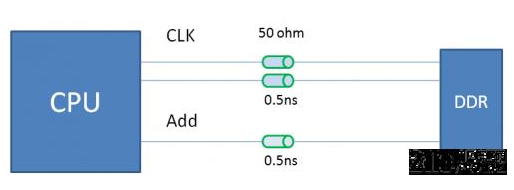Figure 1 Address / Clock Emulation

For the convenience of calculation, we assume that the clock frequency of DDR is 500MHz, so the corresponding address signal speed should be 500Mbps. Here everyone should understand that although DDR is double speed, it is still single speed for address / control signal. Rate. Let's take a look at the waveform. In the case where the address and the clock are exactly the same length, the received waveforms at the address and the data end are shown in Figure 2 below. Red represents the address signal and green represents the clock signal.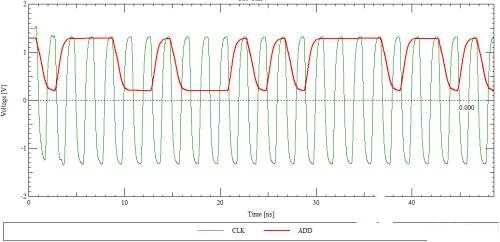Figure 2 Clock signal and address signal waveform

In the above waveform, we don't seem to see what the timing relationship between the clock and address looks like. We put it in an eye diagram, and the timing relationship is very clear. Here we roughly calculate the setup time and hold time. As shown below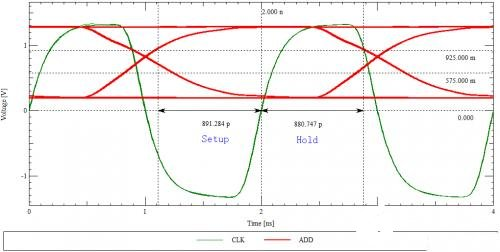Figure 3 Clock signal and address signal waveform

From the above figure 3. we can know that the setup time of this address signal is about 891ps and the hold time is 881ps. This is the waveform when the clock and address signals are exactly the same length. If the address is not equal to the clock, what is the signal like? In the simulation, we made the address line 200ps slower than the clock line, and the resulting eye diagram is as follows: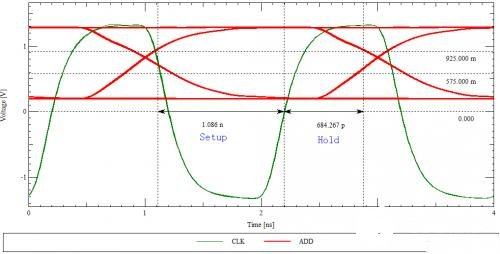Figure 4 Clock signal and address signal waveform

As can be seen from the above figure, when the address signal is longer than the clock signal, the hold time is 684ps, and the establishment is 1.1ns. It can be seen that, relative to the length of the address line and the clock line, the longer the address line is than the clock line, the shorter the setup time of the address signal. Similarly, if the clock line is longer than the address line, the setup time will be longer and the hold time will be shorter. What about a double-rate data signal? Let's look at it through a specific simulation example.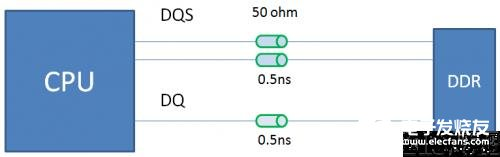Figure 5 DQ and DQS simulation

The simulation channel is shown in the figure above. The driver and receiver are IBIS models of a chip company. The simulation waveform is as follows: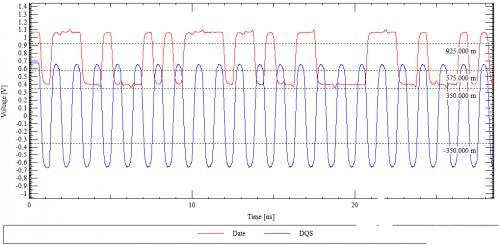Figure 6 DQ and DQS simulation waveforms

We generate the eye diagrams of the DQS and DQ signals at the same time and observe under a window. The results are as follows: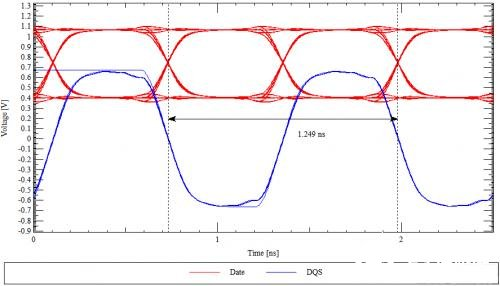Figure 7 DQ and DQS eye diagrams

As shown in the figure above, you may find that if the original correspondence relationship, the edge of the data signal and the edge of the clock signal are aligned. If so, how does the clock signal complete the sampling of the data signal? This is actually not the case. The above simulation simply puts the two waveforms together, because the transmission channels of DQ and DQS have the same length, so their edges are aligned. In actual work, the main control chip will have an adjustment mechanism. Generally, the data signal will be released one quarter cycle earlier than DQS. In fact, the corresponding relationship of the waveform received at the particle end should be like this: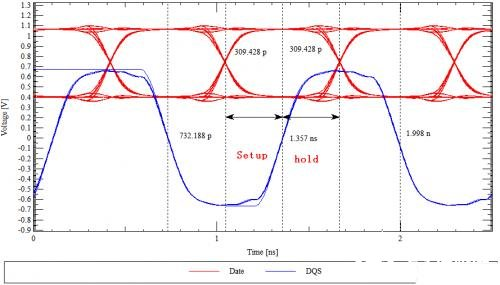Figure 8 Eye diagram after translation

After the adjustment by the main control chip, the edge of the DQS is aligned with the center of the DQ signal bit, so that the data can be transmitted to the receiver with sufficient setup time and hold time. As with the analysis of the clock and address signals above, if the equal length between DQ and DQS is not done well, the clock edge of DQS will not remain in the middle position of DQ, so the margin of setup time or hold time will become smaller.

First look at a picture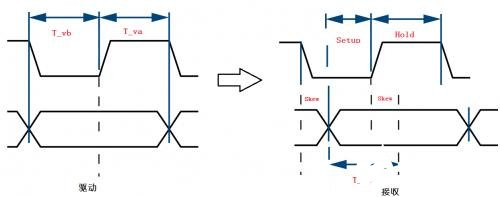Figure 9 Effect of delay deviation on timing

In the figure above, T_vb and T_va represent the timing parameters between the clock and data when the main control chip outputs data. Ideally, the center of the clock edge and the data level are aligned. Since the clock and data transmission channels are not equal in length, the clock edge is not aligned with the middle position of the data pulse, making the margin of settling time smaller. After understanding these basic issues, all we need to do is convert these time parameters into line lengths.

Below we look at the calculation of timing through specific examples. The following figure is the Freescale MPC8572 DDR master chip manual. This picture defines the phase relationship between DQS and DQ when coming out of the chip.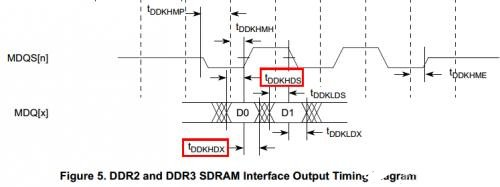Figure 10 MPC8572 timing diagram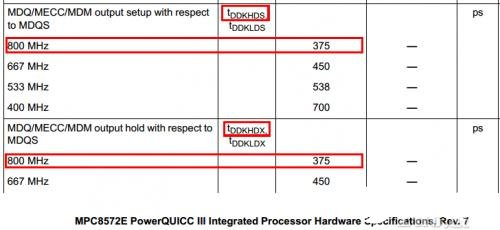Figure 11 MPC8572 timing parameters

The particle end is Micron DDR. The timing diagram and timing parameters of the chip are shown in the following figure. This picture defines the setup time and hold time required for the particle end chip identification signal.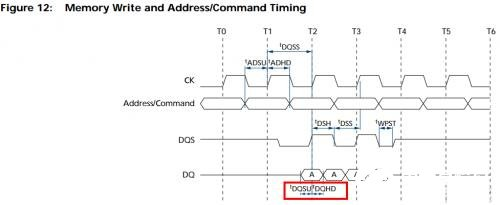Figure 12 DDR particle timing diagram and timing parameters

We use T_pcbskew to represent the delay deviation between DQ and DQS. If we want to get enough timing margin, the delay deviation must satisfy the following relationship:

T_pcbskew < T_vb-T_setup

T_pcbskew > T_hold-T_va

Substituting data, there are:

T_vb-T_setup = 375-215 = 160ps

T_hold-T_va = -160ps

In this way, if the speed of the transmission line is calculated at 6mil / ps, T_pcbskew is +/- 960mil. Everyone will find that there is a lot of margin. Of course, this is only the ideal situation. The jitter of clock and data signals, and the effects of crosstalk and intersymbol interference are not considered. If these factors are taken into account, we will leave the wiring deviation The margin is relatively small.